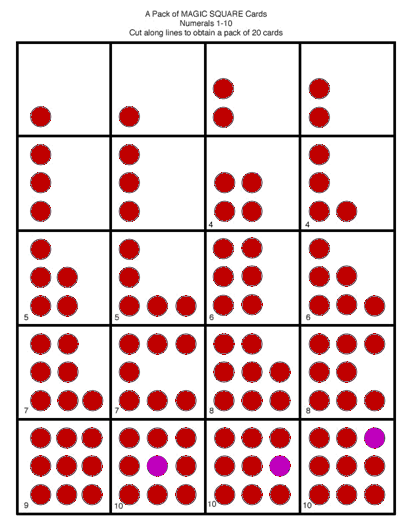# The Maths Square

### The Maths Square – A Fresh Way Forward

Allan Lawson has devised a system of dynamic dot pattern maths that he believes improves teaching methods for addition and subtraction

Why are so many children still failing in numeracy skills? I believe one of the causes lies with the use of rote memory in the early years of maths education. Childrenʼs natural ability to visualise and mentally manipulate patterns can change things.

### Banish rote memory!

The use of rote memory is both an inadequate and an inappropriate teaching method in maths. Yet rote memory is still often relied upon to learn the 55 addition facts up to 10 + 10, and the further 55 multiplication facts in the same range. Thatʼs a total of 110 facts – quite a task for young children – enough to put anyone off maths for good!

I believe rote memory should be left to language teachers, and for learning poetry.  But removing rote memory from early arithmetic is not easy: for what other methods are there enabling pupils to recall the 110 addition and multiplication facts?

And is instant recall even necessary?

### Domino inspired solution

Around 40 years ago, back in the 70’s, when teaching arithmetic to children, I noticed how easily quite young children (4 or 5 years of age) could identify the dot pattern groupings on domino pieces. This lead to a method enabling children to carry out additions and subtractions in the range of natural numbers from 1 to 18 by identifying the numerical value of combined or remaining dominoes. They had natural subitising abilities.

Could dominoes be used effectively in teaching arithmetic at primary level ?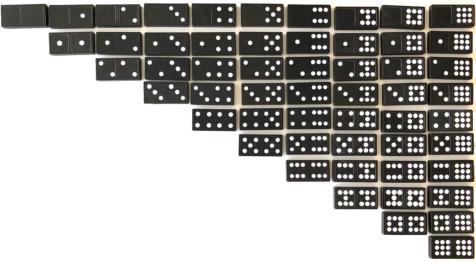### Limits in Subitising domino patterns

In this paper, the author promotes a teaching method for primary mathematics employing a development of Piaget’s subitising of dot patterns: namely the ability of pupils to instantaneously recognise and know the number of dots in a small group without the need to count them.

If such a teaching method is to be adopted, then dominoes have limitations. The first is the limit to the number values that dominoes can show.

Unless we develop domino patterns for use as a numeral system (discussed later) the traditional numerical values given to the highest-value domino, the double 9, is 18. Thus sums which total more than 18 will result in dot patterns not represented by any existing single domino and will form a novel pattern. Novel patterns are those that are beyond the range in numerical value of the fixed stock of 55 dominoes. Thus, until pupils learn to recognise such patterns, there will be errors when attempting to subitise them. The common belief is that most people can only subitise dot patterns up to a group of about 7 – young pupils, less.

But there are more severe problems in using dominoes, even for sums totaling no more than 20 – the sheer volume of dot pattern combinations.

Let us consider using just 2 dominoes to make a combined sum of 19. Limiting the range of numbers available using 2 dominoes, there are 9 combinations of 2 numbers summing to 19, namely

 1 + 18 6 + 13 2 + 17 7 + 12 3 + 16 8 + 11 4 + 15 9 + 10 5 + 14

Now let us consider the number of combinations of 2 dominoes that can be used in each of these sums.

The first sum, 1 + 18, can only be represented by just 2 dominoes :-Next, 2 + 17 can be represented by 2 combinations of 2 dominoes :-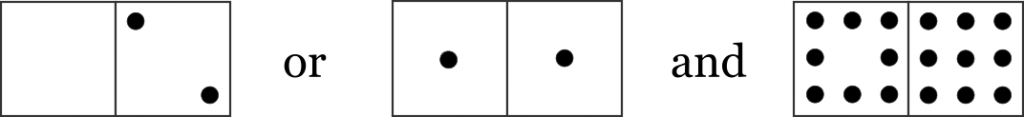Next, 3 + 16 has 4 combinations of 2 dominoes :-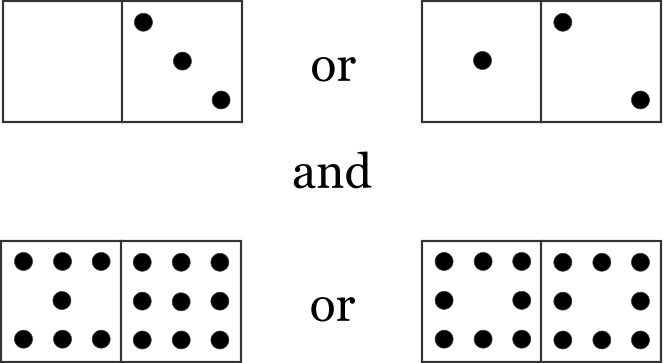Next, 4 + 15 has 6 combinations of 2 dominoes :-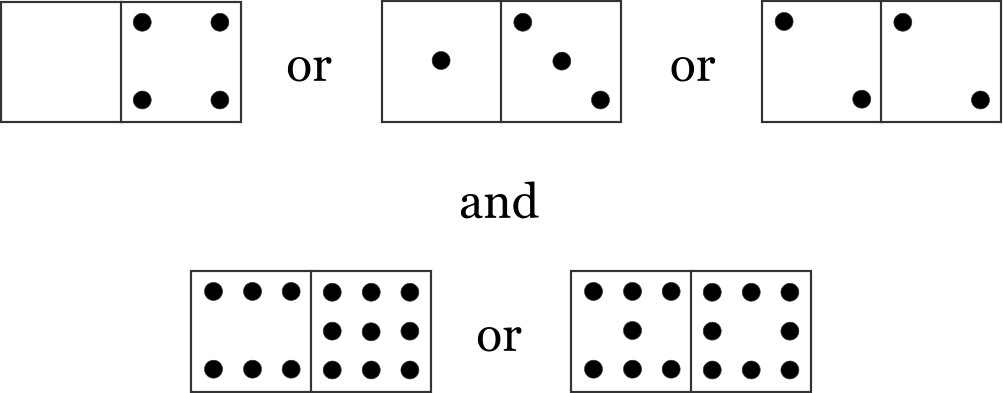5 + 14   has 9     combinations 6 + 13        “ 12          “ 7 + 12        “ 16          “ 8 + 11        “ 20         “ 9 + 10        “ 25         “

The total number of combinations of 2 dominoes to represent the number 19 is thus 95.

Similarly there are 9 combinations of 2 numbers less than 19, that add to 20 :-

 2 + 18    has    2   combinations of 2 dominoes 3 + 17       ”      2                “ 4 + 16       ”      6                “ 5 + 15       ”      6                “ 6 + 14       ”      12              “ 7 + 13       ”      12              “ 8 + 12       ”      20             “ 9 + 11        ”      20             “ 10 + 10      ”      25             ” Total:             105  combinations of 2 dominoes

These numbers of combinations of just 2 dominoes to represent such small additions, are truly daunting if required to be memorised and hence subitised.

### Dominoes – as a numeral system ?

Dominoes were never meant to be a numeral system. The above results show this. But they could be.

Given the following ideas, first evolved in ancient times, dominoes too, could be made to represent any number, and thus become a complete numeral system. All that is needed is a sense of place value, such as base 10. Also a reading instruction: read the two dot patterns on a domino as two separate numbers. In a row of several dominoes, the extreme right-hand number has its face value multiplied by 10 to the power 0, the number to its left, has its face value multiplied by 10 to the power 1; the next, multiplied by 10 to the power 2, and so forth, providing the place value, base 10, to each number. And finally, a row of dominoes should be read from left to right.

So, for example : –

93,806,230

Could be represented in dominoes, thus : –Where the number of digits is odd, such as : –

9,380,623

The first domino on the left, must show 0 on its left-hand side : –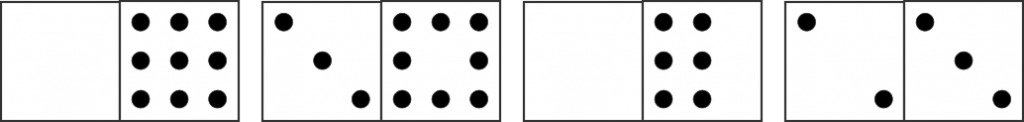So a domino numeral system could easily be developed.

It would, however, be necessary to have  several sets of dominoes to represent certain numbers, especially where a series of the same numbers recurred, for example : –

93,000,000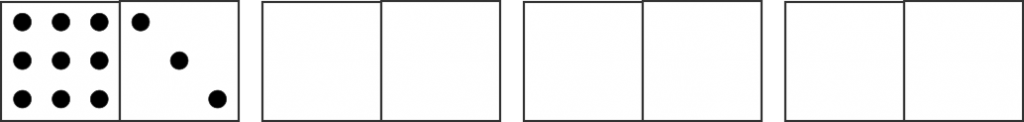In this example, 3 double zero dominoes would be needed to represent the 6 zeros – should physical dominoes be used. Once we move into using diagrams, instead of physical dominoes, the problem disappears.

### Domino mathematics

The Romans developed a fine numeral system, used to this day – but was it of any use in conducting arithmetic? We know it was not. For small numbers, say 2 (Arabic) digit numbers, no doubt rote memory must have been used to obtain each number fact. But for larger numbers carrying out arithmetic operations required a calculator: the abacus. Once a calculation had been completed, the result had to be converted back into Roman numerals, if it was to be expressed in writing on slate, paper or on monuments.

Would dominoes present the same difficulties ? Let us try an example. Multiply 63 by 89 : –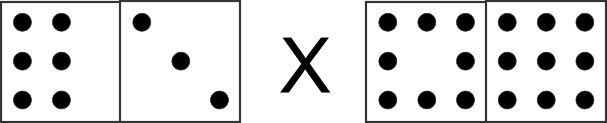The problem could be solved using the traditional algorithm, where rote memory is used in each step of the solution. So there would be no real difficulties.

How about a division ? Lets try : –So, once again, no substantial difficulties.

Although dominoes could, of course, be used to teach arithmetic at primary level, I felt that a better system could be devised, perhaps modeled on dominoes. But my instincts told me that dominoes had further limitations, especially when moving on from addition and subtraction to multiplication and division.

### The Math Square – a dot- pattern numeral system

The limitations that dominoes presented acted as an incentive for me to create new dot patterns which could be more useful in carrying out arithmetic. I decided upon a systematic set of dot patterns to represent numbers to 20 – rather than a set of random dots that werenʼt in some form of pattern – to cover all additions to 20.

Whatever new numeral system was to be created using dot patterns, there was no question that it had to be decimal based. Then, similar to dominoes, a series of new dot patterns could be created to represent the set of 9 unit natural numbers and the number ten. As with dominoes, the patterns would be positioned inside a rectangle or square. A square without any dots would represent zero.

I considered various geometrically-pleasing matrices or “place-settings” for the initial 10 natural numbers. Some of the rejected ones included the following arrangements : –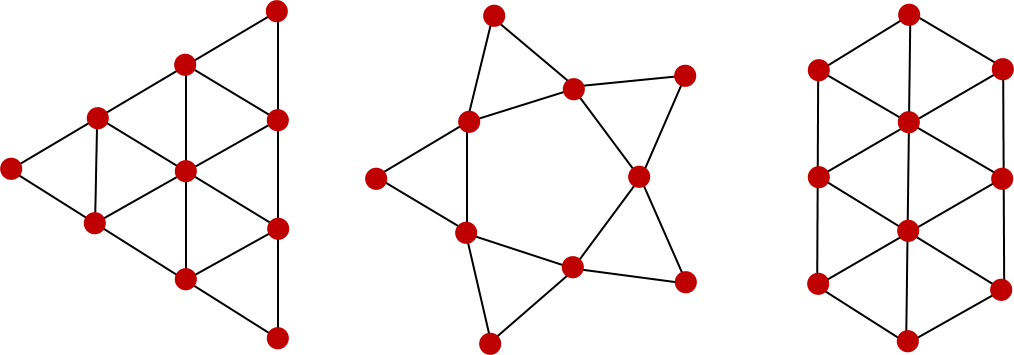I settled on, perhaps, an unlikely format for a 10-based matrix, namely a 3-by-3 square containing 9 locations where dot pattern numerals could be positioned.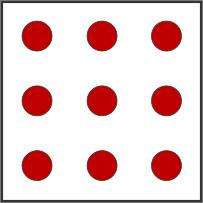I set out some rules governing acceptable spots where dots could be placed to form number patterns : –

1) Dots can only be placed in the 9 designated fixed positions within the square.

2) All dots should be the same colour, shape and size, with the exception of a tenth dot (explained later).

3) No dot pattern should exceed 10 dots in any square.

4) Each dot must be positioned horizontally or vertically next to another dot, unless a single dot is to be used in a square to represent the number 1.

5) No dot should be positioned diagonally to another dot, unless it is also next to another dot horizontally or vertically.

———————————————————–

My reason for setting such rules was to limit the possible number of dot patterns that could be created for each number, thereby reducing the total number of patterns representing the numbers from 1 to ten, down to 67 different patterns. However, a basic set of dot patterns that pupils would need to learn to recognise would be no more than ten.

My base set of patterns to represent numbers 1 to 9 were : –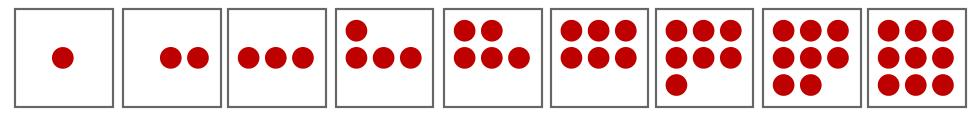This numeral system, as yet, lacked a dot pattern for the number 10. An obvious problem faced me: where, in a 9-dot format, was a tenth dot to be placed in accordance with the above rules?

The problem was solved by my young primary-level pupils when this question was posed to them as they played with a set of dot-pattern cards and some loose plastic counters (or “chips”, as they are sometimes called).

And so this leads me to my choice of suitable material items. Loose red counters were my first choice and they proved useful when dealing with small numbers, say 5 or below.  But using counters becomes cumbersome when doing maths with larger numbers. So square white cards with stick-on red dots were introduced and proved very practical when used with red counters of roughly the same size and colour as the red dots on the cards.

Using these materials, lead to the solution of the problem above: how to represent the number ten using a 9-dot place-setting square ? With the 9 fixed positions, where could a tenth dot or counter be placed?One answer would be to have it placed in another, new square, representing tens; the first square used solely for representing the unit numbers. However, the problem was deftly solved another way, when a small hand picked up a red counter and, without hesitation, placed it in the square on top of the centre dot of a 9-dot pattern.

And thus, we arrived at a 10-place format for expressing number, which I initially named the “Magic Square” but later re-named the “Maths Square”. Some amateur maths teachers I showed this to in the following years liked the name “Magic Square” and have continued to use this term, or similar, such as “Magic Blackboard”.

However, another problem emerged later when representing the Math Square in diagrammatic form.

Whilst using red counters alone to express the number 9, and wanting to add a dot to form the number 10 by placing a counter on top of the centre counter, the newly created 10-dot pattern could readily be distinguished from the earlier 9-dot pattern. On close examination, the centre position with two counters was obvious and visible. But what if diagrams alone were to be used ? It would be impossible to distinguish a 9-dot pattern from a 10-dot pattern using dots of the same size, colour and shape.

The solution was to treat the tenth dot as “special”, by awarding it its own colour – purple.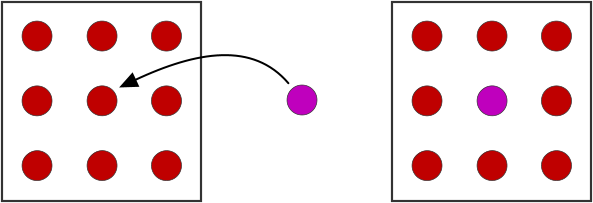The purple dot now indicates that there are two counters or dots in the position where a purple dot is positioned. Thus, where the other 9 places in the Math Square are filled with dots or counters, we have a 10-dot pattern.

In a later development of this numeral system, a purple dot alone would express the number 10.

Then red dots would be banished from sharing a square with a purple dot or dots. Each purple dot would represent the number 10 and the dot-pattern numeral system would apply to squares containing purple dots. Purple dot patterns in their own Math Square would represent numbers raised by 10 to the power 1.The pattern above shows ten represented by a dot pattern in a Math Square. Later, we shall see alternatives, where the tenth purple dot can be positioned elsewhere in the ten dot pattern.

Numbers between 10 and 20 were arranged on cards double in size, with a 10-pattern on the left-hand half of the card and patterns from 1 to 10 arranged on the right-hand side.

Before using the new cards with children, I used only plastic counters to arrange the dot patterns in order to provide plenty of hands-on activity, with pupils adding or taking away counters from various pattern arrangements in doing sums.

As sums of 5 + 5 and higher were approached, it became useful to use a combination of Math Square cards and plastic counters. The dots printed on the cards were made in the same colour and size as the loose plastic counters. Counters can then be placed on the cards to create new dot patterns in addition or dots covered with white card in subtraction.

### Variations of patterns for some numbers

For some of the numbers, such as 4 or 5, I found that two or more variations of patterns were found to be useful in carrying out additions. Below are patterns that teachers may wish to use: they are not exclusive and teachers may find other patterns more useful (See Appendix A).

The essential point I found is this: in order for children to re-assemble and re-arrange dot patterns into other identifiable dot patterns, a dot pattern numeral system must already be established in their minds, no matter what dot patterns are used. Once a set of dot pattern numerals has been chosen, it must be memorized by pupils. The use of flash cards will help. Here are my suggestions :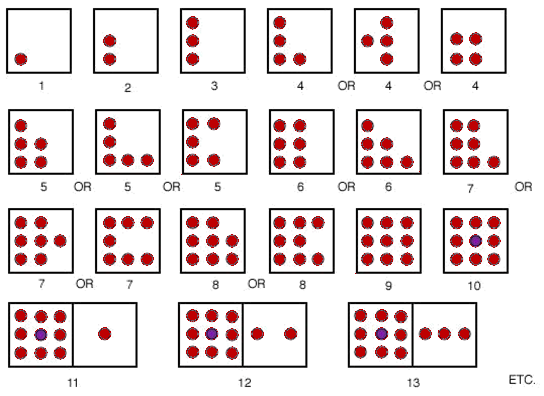The patterns should be identifiable from any orientation, whether as mirror images or revolved images as this will help pupils in performing dot pattern arithmetic.

In performing additions of two numbers, the aim is to merge or re-arrange and then re-assemble the 2 dot patterns to form a single identifiable new pattern. Where it seems possible to create a 10 pattern, this must be the first step. Making a 10 pattern is essential knowledge, as it is when using, say, Dienes blocks, an abacus or any other decimal system.

I suggest below some strategies to assist in creating a 10 pattern where one of the numbers in the addition sum is a 6, 7, 8 or 9. These numbers can be “built up” to form 10 by removing dots from the other number “n” in the addition.

So, where we have (6 + n), n being in the range 1 to 4, the aim is to build up the 6 dot pattern by attaching the n pattern to the 6 pattern, or where n ≥ 5, by removing 4 dots from the n pattern, attaching them to the 6 pattern, thus forming a new 10 pattern. The resulting pattern formation will then be in identifiable form.

Below is displayed a single strategy for the solution of each example addition:

The many equally good alternatives for re-arranging and re-assembling the dot patterns are left for you and your pupils to explore.

i) 6 + 3 : the 6 and 3 patterns merge to make the 9 pattern: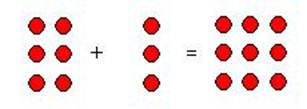ii) 6 + 4 : the 6 and 4 patterns merge to make the 10 pattern: in the process of merging, two dots overlap at the center of the square – and is represented by a purple dot. Only a single overlap is allowed in any ten dot square. Overlapping dots are not allowed in smaller numerals.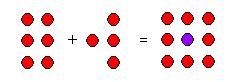iii) 6 + 5 : in dot patterns :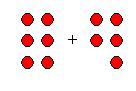Before merging, the 5 pattern can be re-arranged as a 4 pattern, plus one: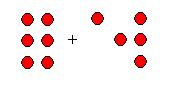Then the 4 pattern can be merged with the 6 pattern to make a 10 pattern, with 1 dot remaining – resulting in the 11 pattern: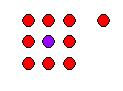iv) 6 + 6 : in dot patterns :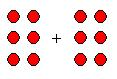Before merging, the right-hand 6 pattern can be re-arranged as a 4 pattern plus a two pattern:Now the 4 pattern can be merged with the 6 pattern, the re-assembled dots making a 10 pattern, with 2 dots remaining; resulting in the 12 pattern: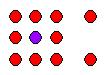In additions of (7 + n) the rule to follow is to remove 3 dots from n, the other number, and merge these with the 7 pattern, so forming a 10 pattern. Eg:-

i) 7 + 3 : the 7 and 3 patterns merge to make another form of the 10 pattern: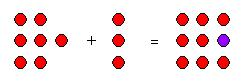ii) 7 + 8 : by removing 3 dots from the 8 pattern and merging these with the 7 pattern, a 10 pattern is formed, with a 5 pattern remaining, the two new patterns forming a 15 dot pattern: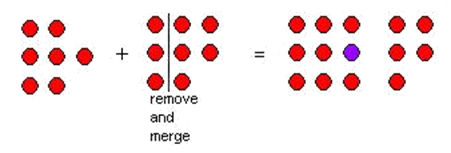In additions of (8 + n), remove the 2 dots from the other number n, and merge these with the 8 pattern, to make a 10 pattern. Eg:

i) 8 + 2 : the 8 and 2 patterns merge to form a 10 pattern: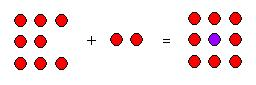ii) 8 + 8 : the suggested steps needed to form a 16 pattern are: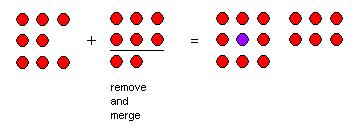In additions of (9 + n), we simply remove one dot from n and merge it with the 9 pattern, making a 10 pattern e.g:

9 + 9 :### 10, 20 and beyond

The Math Square dot pattern numeral system does not stop at 20. However, to reduce the number of dots required when dealing with larger numbers, we could dispense with some of the red unit dots for all numbers larger than 9. So instead of 10 consisting of 9 red dots and a central purple dot, it could be represented by the purple dot alone. And 20 would be represented by two purple dots, thirty by 3 purple dots, and so on. One hundred could be represented by a third coloured dot, say yellow, with each successive power of ten being represented by yet another colour. For example 1,342 might be represented as: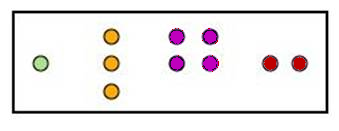The Math Square numeral system could in theory represent enormous numbers, limited only to the extent that colours might be exhausted in representing higher and higher powers of ten. And in such a system, where colour denotes decimal power, the position of each coloured pattern is immaterial.

In a future article, I will describe how the Math Square was developed into an ordinal place-value numeral system capable of representing all positive whole numbers to infinity – and beyond!

### Subtraction

I have not provided examples of how subtraction can be performed. But teachers can experiment with the dot pattern cards, in a similar way as some additions are carried out, by the removal of counters from a dot pattern or by covering up dots on cards to show the resulting dot pattern in an identifiable form.

### Mental arithmetic

One remarkable development that came to light from children who had played with the dot pattern cards and counters for some while was their ability to visualize the dot patterns in their mindʼs eye, then to re-arrange and re-assemble them into identifiable patterns, without the use of any physical materials.

### Before I conclude: Q – Why have an overlapping tenth dot?

A criticism that could be leveled at this numeral system is this: wonʼt an overlapping tenth dot in the 10-dot pattern cause confusion with the 9-dot pattern? Indeed, why introduce a dot numeral system with an overlapping tenth dot? After all, there are other regular 10-dot patterns without overlapping dots that can form the basis of a numeral system. For example: –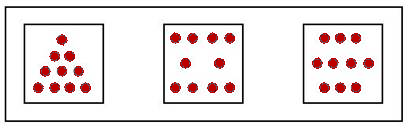However, my justification in choosing the Math Square format lies with itʼs development into a similar square with a tenth position lying at itʼs centre, for use in solving the multiplication facts. The tenth overlapping central position becomes vital in this development.

### Conclusions

1. Childrenʼs visual mental ability:

Children can easily identify dot pattern numbers; they can also visualize dot patterns in their mindʼs eye and re-arrange and re-assemble these patterns to form previously known patterns. And they have no difficulty in distinguishing the 9-dot pattern from the 10-dot pattern.

The Math Square system provides children with good opportunities to exercise their visual mental ability to manipulate dot patterns, and a fresh way to explore the possibilities to be discovered in dot pattern maths.

In my opinion psychologists should re-examine the role that childrenʼs visual mental ability could play in the teaching of mathematics generally.

2. Memorizing facts unnecessary:

Employing these methods to carry out additions and subtractions has shown me that committing the facts to memory for instant recall is unnecessary. Children can quickly arrive at the results of the 55 additions mentally using this system of dynamic dot pattern maths.

### Next

The following article entitled The Decimal Square shows how the Math Square format was developed into a positional framework with vectors enabling children to find the facts of the multiplication tables mentally in a systematic way in place of rote memory. Visualized spatial abilities are here too employed.

For those who wish to try some Math Square cards, the on-line version of this article has a printable page of cards, which can be cut or guillotined to provide a set of 20 cards.